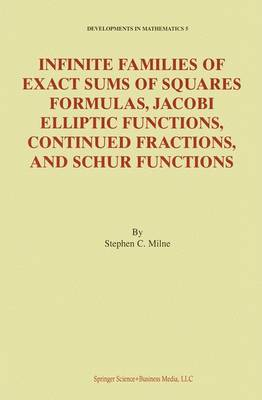•Infinite Families of Exact Sums of Squares Formulas, Jacobi Elliptic Functions, Continued Fractions, and Schur Functions - Developments in Mathematics 5 (Paperback)

(author)
£89.99
Paperback 143 Pages / Published: 05/01/2011
• We can order this

Usually dispatched within 3 weeks

The problem of representing an integer as a sum of squares of integers is one of the oldest and most significant in mathematics. It goes back at least 2000 years to Diophantus, and continues more recently with the works of Fermat, Euler, Lagrange, Jacobi, Glaisher, Ramanujan, Hardy, Mordell, Andrews, and others. Jacobi's elliptic function approach dates from his epic Fundamenta Nova of 1829. Here, the author employs his combinatorial/elliptic function methods to derive many infinite families of explicit exact formulas involving either squares or triangular numbers, two of which generalize Jacobi's (1829) 4 and 8 squares identities to 4n2 or 4n(n+1) squares, respectively, without using cusp forms such as those of Glaisher or Ramanujan for 16 and 24 squares. These results depend upon new expansions for powers of various products of classical theta functions. This is the first time that infinite families of non-trivial exact explicit formulas for sums of squares have been found.

The author derives his formulas by utilizing combinatorics to combine a variety of methods and observations from the theory of Jacobi elliptic functions, continued fractions, Hankel or Turanian determinants, Lie algebras, Schur functions, and multiple basic hypergeometric series related to the classical groups. His results (in Theorem 5.19) generalize to separate infinite families each of the 21 of Jacobi's explicitly stated degree 2, 4, 6, 8 Lambert series expansions of classical theta functions in sections 40-42 of the Fundamental Nova. The author also uses a special case of his methods to give a derivation proof of the two Kac and Wakimoto (1994) conjectured identities concerning representations of a positive integer by sums of 4n2 or 4n(n+1) triangular numbers, respectively. These conjectures arose in the study of Lie algebras and have also recently been proved by Zagier using modular forms. George Andrews says in a preface of this book, `This impressive work will undoubtedly spur others both in elliptic functions and in modular forms to build on these wonderful discoveries.'

Audience: This research monograph on sums of squares is distinguished by its diversity of methods and extensive bibliography. It contains both detailed proofs and numerous explicit examples of the theory. This readable work will appeal to both students and researchers in number theory, combinatorics, special functions, classical analysis, approximation theory, and mathematical physics.

Publisher: Springer-Verlag New York Inc.
ISBN: 9781441952134
Number of pages: 143
Weight: 251 g
Dimensions: 235 x 155 x 8 mm
Edition: Softcover reprint of hardcover 1st ed. 2002